# JS的深浅拷贝 ​

## 数据类型 ​

• 基本数据类型的特点：直接存储在栈(stack)中的数据
• 引用数据类型的特点：存储的是该对象在栈中引用，真实的数据存放在堆内存里## 浅拷贝与深拷贝 ​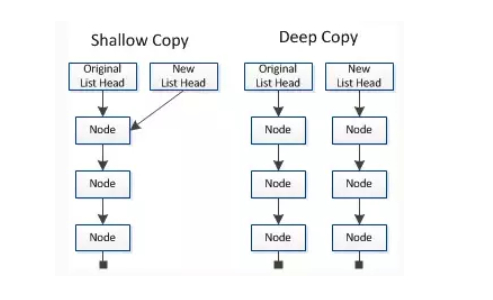## 赋值和浅拷贝的区别 ​

1. 当我们把一个对象赋值给一个新的变量时，`赋的其实是该对象的在栈中的地址，而不是堆中的数据`。也就是两个对象指向的是同一个存储空间，无论哪个对象发生改变，其实都是改变的存储空间的内容，因此，两个对象是联动的。
1. 浅拷贝是按位拷贝对象，`它会创建一个新对象`，这个对象有着原始对象属性值的一份精确拷贝。如果属性是基本类型，拷贝的就是基本类型的值；如果属性是内存地址（引用类型），拷贝的就是内存地址 ，因此如果其中一个对象改变了这个地址，就会影响到另一个对象。即默认拷贝构造函数只是对对象进行浅拷贝复制(逐个成员依次拷贝)，即只复制对象空间而不复制资源。

js
``````// 对象赋值
var obj1 = {
name: "zhangsan",
age: "18",
language: [1, [2, 3], [4, 5]]
};
var obj2 = obj1;
obj2.name = "lisi";
obj2.language = ["二", "三"];
console.log("obj1", obj1);
console.log("obj2", obj2);``````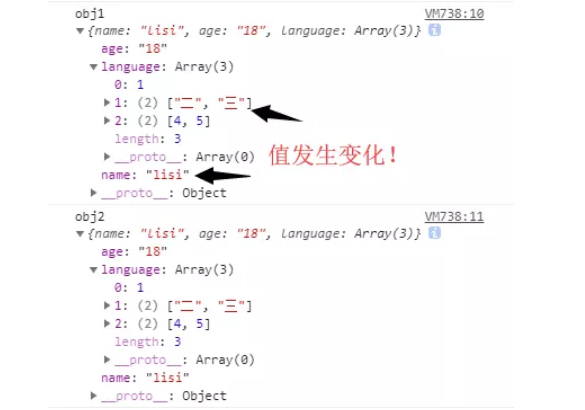js
``````// 浅拷贝
var obj1 = {
name: "zhangsan",
age: "18",
language: [1, [2, 3], [4, 5]]
};
var obj3 = shallowCopy(obj1);
obj3.name = "lisi";
obj3.language = ["二", "三"];
function shallowCopy(src) {
var dst = {};
for (var prop in src) {
if (src.hasOwnProperty(prop)) {
dst[prop] = src[prop];
}
}
return dst;
}
console.log("obj1", obj1);
console.log("obj3", obj3);``````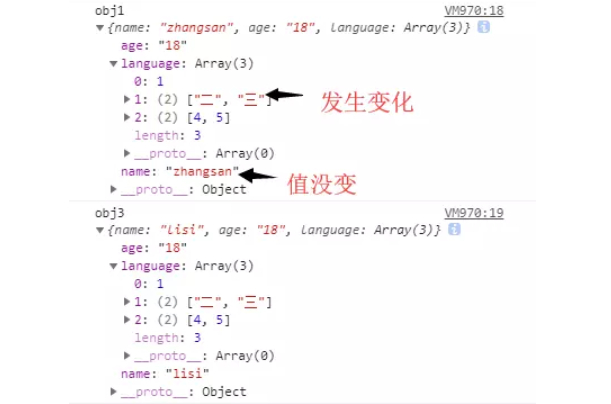/和原数据是否指向同一个对象第一层数据为基本数据类型原数据中包含子对象

## 浅拷贝的实现方式 ​

### 1.Object.assign() ​

Object.assign() 方法可以把任意多个的源对象自身的可枚举属性拷贝给目标对象，然后返回目标对象。但是 Object.assign()进行的是浅拷贝，拷贝的是对象的属性的引用，而不是对象本身。

js
``````var obj = { a: { a: "kobe", b: 39 } };
var initalObj = Object.assign({}, obj);

js
``````let obj = {
};
let obj2 = Object.assign({}, obj);

### 2.Array.prototype.concat() ​

js
``````let arr = [
1,
3,
{
}
];
let arr2 = arr.concat();
console.log(arr);``````### 3.Array.prototype.slice() ​

js
``````let arr = [
1,
3,
{
}
];
let arr3 = arr.slice();
console.log(arr);``````Array 的 slice 和 concat 方法不修改原数组，只会返回一个浅复制了原数组中的元素的一个新数组。

• 如果该元素是个对象引用(不是实际的对象)，slice 会拷贝这个对象引用到新的数组里。两个对象引用都引用了同一个对象。如果被引用的对象发生改变，则新的和原来的数组中的这个元素也会发生改变。
• 对于字符串、数字及布尔值来说（不是 String、Number 或者 Boolean 对象），slice 会拷贝这些值到新的数组里。在别的数组里修改这些字符串或数字或是布尔值，将不会影响另一个数组。

js
``````let arr = [
1,
3,
{
}
];
let arr3 = arr.slice();
arr3 = 2;
console.log(arr, arr3);``````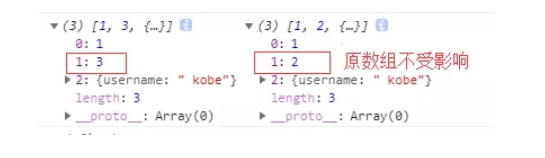## 深拷贝的实现方式 ​

### 1.JSON.parse(JSON.stringify()) ​

js
``````let arr = [
1,
3,
{
}
];
let arr4 = JSON.parse(JSON.stringify(arr));
console.log(arr, arr4);``````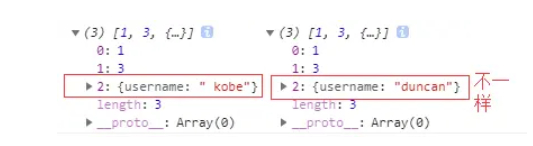js
``````let arr = [
1,
3,
{
},
function() {}
];
let arr4 = JSON.parse(JSON.stringify(arr));
console.log(arr, arr4);``````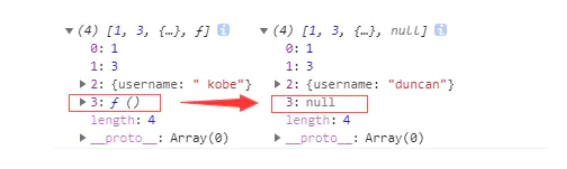### 2.手写递归方法 ​

ts
``````function deepCopy(from: any, map: any = new Map()) {
const isObject = (obj: any): obj is Object =>
Object.prototype.toString.call(obj) === "[object Object]" ||
Object.prototype.toString.call(obj) === "[object Array]";

if (!isObject(from)) return from;
let res: any = Array.isArray(from) ? [] : {};

// 有缓存时不用递归，解决循环引用问题
if (map.get(from)) res = map.get(from);
else {
// 缓存当前对象
map.set(from, res);
for (const key of [
...Object.keys(from),
...Object.getOwnPropertySymbols(from),
]) {
const val = from[key];
if (isObject(val)) {
res[key] = deepCopy(val, map);
} else {
res[key] = val;
}
}
}
return res;
}``````

js
``````let a = {
age: 11,
arr: [1, 2, 3],
say: function() {},
m: Symbol(1)
};
const b = deepCopy(a)
b.age = 12
b.arr = 4
b.m = Symbol(2)
console.log("a", a)
console.log("b", b)
// 输出
a { age: 11, arr: [ 1, 2, 3 ], say: [Function: say], m: Symbol(1) }
b { age: 12, arr: [ 1, 4, 3 ], say: [Function: say], m: Symbol(2) }``````

### 函数库lodash ​

js
``````var _ = require('lodash');
var obj1 = {
a: 1,
b: { f: { g: 1 } },
c: [1, 2, 3]
};
var obj2 = _.cloneDeep(obj1);
console.log(obj1.b.f === obj2.b.f);
// false``````